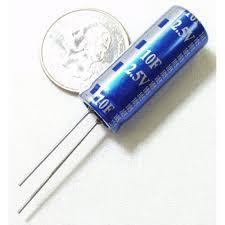# Educational Post: Capacitors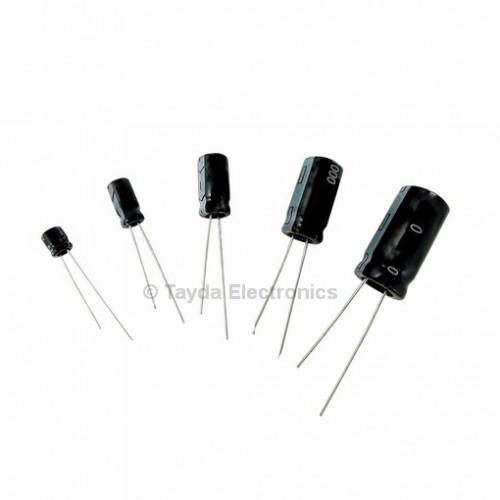Capacitors, they are often used to smooth power supplies or signals, they also have a huge variety of other uses from blocking DC currents and allowing AC signals.

The basic idea behind a capacitor is that it is a power storage device. A capacitor temporarily stores electrical energy in an electric field.

Capacitors use two conductors on either side of a nonconducting material. The conductors are generally thin films, foils, or sintered beads of conducting material. This is seperated by many things, glass, ceramic, plastic, paper, mica, or just about anything that will not conduct electricity.

When a capacitor is connected to a DC power source, a battery usually, a charge builds up on one conducting plate. As this charge builds on the positive side plate the negative side plate collects negative voltage. Unlike resistors ideal capacitors do not dissipate energy.

The larger the plates and the smaller the space between the two plates the larger the capacitance. In practice there is some leaking current over the insulating material.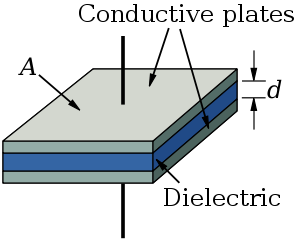The capacitance varies greatly based on the distance between the two conductors. The larger the plates the larger the capacitance, while the closer the plates the higher the capacitance. The total capacitance of a capacitor can be calculated by the following equation.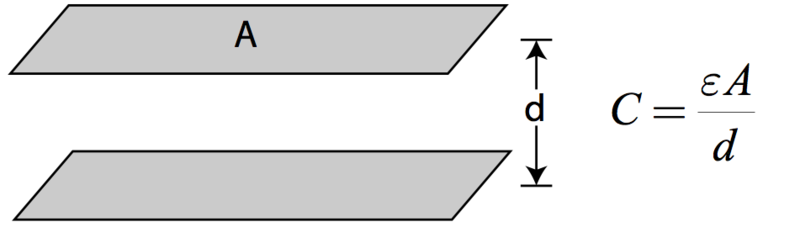In this case A is the area of the parallel plates. Epsilon is the permitivity of free space, it's a constant. Divided by the distance between the two plates.

Capacitance is measured in Farads. 1 Farad is equal to 1 Amp * Second over a Volt. When a voltage changes at 1 Volt/Second a Current of 1 Amp results.

There are many different types of capacitors. The list includes:

Ceramic Capacitors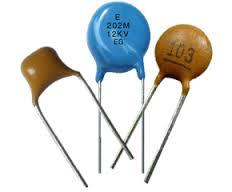Aluminum and Tantalum Capacitors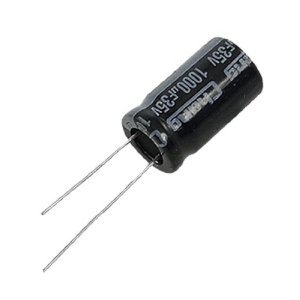Film Capacitors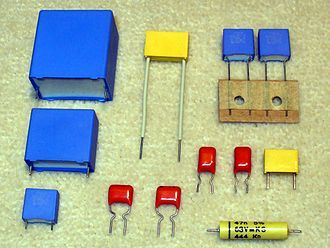Super Capacitors